Reach Us+44-1522-440391
Modeling the Log Density Distribution with Orthogonal Polynomials | OMICS International
Journal of Biometrics & Biostatistics

Like us on:

All submissions of the EM system will be redirected to Online Manuscript Submission System. Authors are requested to submit articles directly to Online Manuscript Submission System of respective journal.

# Modeling the Log Density Distribution with Orthogonal Polynomials

Eugene Demidenko*

Dartmouth Medical School, Section of Biostatistics and Epidemiology, New Hampshire, USA

*Corresponding Author:
Eugene Demidenko
Dartmouth Medical School
Section of Biostatistics and Epidemiology
7927 Rubin Building, One Medical Center Drive
Lebanon, NH 03756, USA
Tel: (603) 653-3682
E-mail: [email protected]

Received Date: October 30, 2010; Accepted Date: November 09, 2010; Published Date: November 12, 2010

Citation: Demidenko E (2010) Modeling the Log Density Distribution with Orthogonal Polynomials. J Biomet Biostat 1:105. doi:10.4172/2155-6180.1000105

Copyright: © 2010 Demidenko E. This is an open-access article distributed under the terms of the Creative Commons Attribution License, which permits unrestricted use, distribution and reproduction in any medium, provided the original author and source are credited.

Visit for more related articles at Journal of Biometrics & Biostatistics

#### Abstract

A possibly multimodal log density is modeled via orthogonal polynomials. An efficient Fisher scoring algorithm for maximum likelihood density estimation is described. Statistical hypothesis testing is emphasized such as the test for normality and density multimodality. The density estimation is illustrated with two biomedical examples: brain oxygen distribution and toenail arsenic distribution among New Hampshire residents.

#### Keywords

Bump hunting; Fisher scoring; Hypothesis testing; Maximum likelihood; Multimodality

#### Introduction

Density estimation is one of the most important and difficult statistical problems. There exists a vast literature on the topic and this is not the goal of the paper to provide an overview of all existing approaches. Mainly three methodologies have been developed: First, a nonparametric approach can be used, such as kernel density estimation, penalized maximum likelihood or spline density smoothing . Second, finite mixture can be applied to model multimodal distributions . Third, polynomials can be used for the log density modelling and estimation. The latter approach, taken in the present paper, is the simplest and can be used at a preliminary stage of density estimation.

The rationale for using polynomials of a higher order for the log density is as follows. First, the log density of the normal distribution is the polynomial of the second order. Second, since polynomials may have several minima and maxima of the higher order they can describe multimodal distributions, as does the nonparametric approach. Third, by testing the statistical significance of the efficients of the polynomial greater than two we can test the hypothesis that the distribution is normal.

We hypothesize that (a) the real data distribution do not have many components, say two or three and (b) the density function is fairly rounded. If so, expressing the density function through a polynomial of order 4 or 6 becomes justifiable. For example, a polynomial of the fourth order may describe a bimodal density pointing out to the presence of a subpopulation with outstanding values. As was mentioned above, the advantage of polynomial density is that it is parametric and therefore classic statistical hypothesis testing applies. For example, to test that the density is Gaussian, we simply test the hypothesis that the coefficients at the powers higher than 2 are zero; to test that the density is unimodal, we test that the polynomial derivative has a unique root. Especially attractive, in the framework of exponential polynomial fitting, is statistical hypothesis testing such as “Is density Gaussian or bimodal?”

Several authors, including  and  used polynomials to model density. Although this approach is computationally straightforward it may produce negative values of the density estimate. Following  and , we use exponential polynomials that guarantees positive density values. An important argument in favor of modeling density via exponential polynomial is the fact that if the order of polynomial is 2 one obtains a normal distribution. Unlike previous authors, we do not use penalization since we advocate a moderate polynomial order. Since our approach is completely parametric, we apply statistical hypothesis testing to chose the order of the polynomial. The goal of the present paper is to concentrate on computationally effective estimation algorithms with relevant hypothesis testing.

The structure of the papers is as follows. In the next section we describe a parametric model for the log density via an orthogonal polynomials. In section 3, we develop the Fisher Scoring algorithm for maximum likelihood estimation. In section 4, we illustrate density estimation with two examples, the rat brain oxygen distribution and toenail arsenic concentration in New Hampshire residents. We show how to statistically test that the distribution is normal.

#### Density Model

We model the log density distribution via a polynomial, or equivalently, the density f via an exponential polynomial of order K ≥ 2 as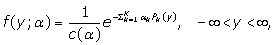(1)

where Pk(y) is a polynomial of the kth order with known coefficients,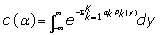(2)

is the normalizing coefficient and α = (α1, ..., αK)' is the parameter vector (boldface is used for vectors and matrices). Note that there is no constant term (k > 0) because it is saturated in the normalizing coefficient. Thus, we assume that observations are continuously distributed on (-∞,∞). In a special case Pk(y) = yk; this parametrization will be called canonical. Alternatively we may call the density model (1) as exponential polynomial.

Since polynomial values are highly correlated, the problem of the coefficients estimation becomes ill-posed. To facilitate computations, orthogonal polynomials have been proposed.There are several systems of orthogonal polynomials on the interval [0,1], for example. Laguerre, Legendre, or Chebyshev, that differ by the definition of the scalar product defined via an integral . Usually, Hermite polynomials are used for density modeling defined on (-∞,∞) with the weight function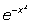. Importantly, coefficients of orthogonal polynomials from different systems are linearly dependent. For example, if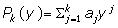with coefficients collected in a k × 1 vector, a, and Hk(y) is Hermite polynomial with the k × 1vector of coefficients, b, then there exist a k ×k nonsingular matrix, M, such that b = Ma. Consequently, different polynomial systems simply imply different linear parametrizations of α in the density estimation (1).

We, however, prefer a data-based definition of polynomial orthogonality, namely,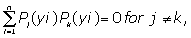where y1, y2, ..., yn are observations. In fact, we expect that the data-based orthogonality would be more efficient because statistical computations are carried out on the sample values,not in the functional space defined on (-∞,∞) as is assumed for Hermite polynomials. Databased orthogonal polynomials are readily available in popular statistical packages such as S-Plus (function poly).

Exponential polynomial density estimation (1) leads to a nonlinear statistical problem that involves integration (2). When K > 2, there is no explicit formula for c(α) and numerical integration is required–this is the major technical problem in estimating parameters of density (1).

#### Maximum Likelihood Estimation

If {yi, i = 1, 2, ..., n} are iid observations from f, the log-likelihood function takes the form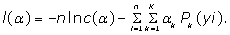In this section, we assume that K is an even known number and αK> 0. These conditions imply that the integral (2) exists. In fact, positiveness and statistical significance of the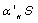estimate may be a guideline for choosing an appropriate K.

Without loss of generality one can assume that observations are ascending, namely, y1y2 ≤ ... ≤ yn. There exists no closed form solution of the integral (2) for K > 2, so we apply numerical integration. Generally, the integral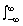G(y)dy is approximated with a sum,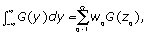(3)

where wq are the weights and {zq, q = 1, 2, ...,Q} is the sample of the argument in the range where the integrand value is not negligible. The easiest way to derive the weights is to take observations themselves as the sample for numerical integration, we refer to this algorithm as the y-value method. Then, from the trapezoid rule, we obtain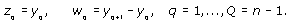(4)

Another way to obtain the sample of z-values is to take equidistant values within the interval (min y - K(max y - min y), max y + K(max y - min y)), where K can be chosen 1/2 or 1/3 to cover the range of possible y-values. Finally, Gauss-Hermite quadrature  applies with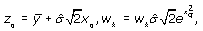where xq and ωk are Gauss- Hermite nodes and weights (these values can be computed using a C-code gauher ).

As a word of caution, numerical integration is a difficult computational problem especially if the integrand function is not unimodal. Although numerical integration may be a building procedure of commercial software we should use it carefully because typically no error analysis is supplied . Also the reader should be aware that integral approximation may not guarantee the desired accuracy unless an analytical investigation of the integrand and its derivatives is carried out, especially when the integration domain is (-∞,∞). A good practice is to reestimate the density with different integration parameters to see whether estimation results are negligibly different. In other words, the sensitivity of estimation results to the choice of Q, zq, and ωq should be addressed in applications. Using approximation (3), we minimize the negative log likelihood function,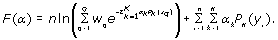(5)

Letting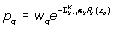the derivatives are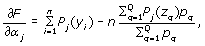(6)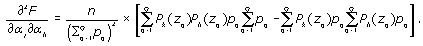(7)

The MLE solves equations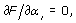j = 1, ...,K. The elements (7) constitute the K×K Hessian matrix H. We prove that this matrix is positive definite. Before that, we rewrite the gradient and the Hessian in matrix form. Let y be the K × 1 vector with components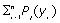, Z be the Q ×K matrix with elements Zqj = Pj(zq) and p be the Q × K vector with components pq. Then the gradient g, defined by (6) and the Hessian H, defined by (7), are written as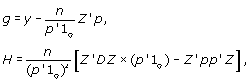where D = diag(p).

We prove that H is a positive-definite matrix. Let u be any nonzero K × 1 vector, then u'Hu is proportional to v'Dv×(p'1Q)-(v'p)2, where v = Z'u. Rewriting this in coordinate form, we have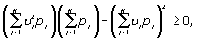as follows from the Cauchy inequality expressing vjpj = vj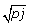The equality holds if and only if, i.e., if vj = const. But Z'u = const would mean that the polynomial of the Kth order has Q roots which is impossible. Thus, the Hessian H is positive definite if K < Q. Consequently, the maximum likelihood estimate is unique because (5) is a strictly convex function. The Hessian, H, is also the Fisher information matrix because H is not random. The inverse, H-1 is the asymptotic covariance matrix of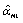, which is used for hypothesis testing. The MLE is found iteratively by the Fisher Scoring algorithm,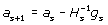(8)

where s = 0, 1, ... is the iteration index and the right-hand side is evaluated at a = as. As the iterations go on, the gradient ||gs|| should vanish.

A good test for numerical quadrature is to run the algorithm with K = 2 and to compare the solution to the exact one. Indeed if K = 2, we obtain the normal distribution with the negative log-likelihood value 0.5n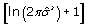, where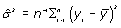. This test may serve as a guide for choosing the right number of nodes.

Initial estimate

To start iterations (8), one has to have an initial guess for the vector of coefficients, a0. The choice of the initial estimate is important because the closer a0 is to the MLE the faster iterations. We suggest the following procedure: Build a histogram with a chosen number of bins, L > K at locations {yl, l = 1, 2, ...,L} and fit -ln pl with {Pk(yl), k = 1, ...,K} using linear least squares, namely, minimizing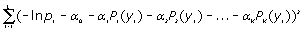(9)

The least squares estimate yields the initial vector a0= (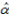1,.....,K )’. Several tries with different numbers of bins may be required to obtain satisfactory estimates, meaning that the coefficient at the highest order polynomial is positive and statistically significant. This procedure may help in determining the right polynomial degree via statistical significance testing of its coefficients.

#### Examples

We illustrate our approach with two examples. The first example has a moderate sample size, n = 270, with evident bimodal distribution, while in the second example, the sample size is fairly large, n = 1057, with a seemingly lognormal distribution. We use the statistical hypothesis approach to test normality and bimodality.

Rat brain oxygen distribution

We use the data from  on the rat brain PtO2 measured with an Eppendorf polarographic electrode device. Knowing the distribution of oxygen in brain is a fundamental biological problem especially in connection with ischemia and lack of oxygen during stroke . The histogram with 25 bins and three methods of density estimation using an exponential polynomial of the sixth order and the Gaussian kernel density is presented in Figure 1. We use the S-Plus built-in function density with the default bandwidth for the density estimation with Gaussian kernel. The bimodality of the brain oxygen distribution is visually obvious.All methods produce somewhat close density curves. The results of estimation are presented in Table 1 (SE are shown below in parentheses). Initial estimation uses linear fit of the sixth degree polynomial to the log frequency values by least squares (9). The nodes in y-value method are the observations themselves and G-H (21) refers to Gauss-Hermite numerical quadrature with 21 nodes. We test the quality of numerical quadrature by running the algorithms with K = 2. For the y-value method, the minimum F value (5) is 1019.52, while for the Gauss-Hermite numerical quadrature with 21 nodes, the value coincides with the exact one, 1033.53. Thus, we infer that the Gauss-Hermite numerical quadrature with 21 nodes yields a precise integral approximation.

 Method αˆ1 αˆ2 αˆ3 αˆ4 αˆ5 αˆ6 m#1 m#2 s.p. Initial (25) -1.256 (0.217) 2.845 (0.217) -0.151 (0.217) 0.489 (0.217) 0.893 (0.217) 0.290 (0.217) 22.7 (1.23) 42.4 (1.34) 35.8 (1.61) y-value -1.315 (1.002) 6.017 (1.004) -1.165 (1.002) 1.192 (1.090) 2.973 (1.152) 0.706 (1.072) 20.6 (1.74) 43.6 (1.37) 35.4 (1.83) G-H (21) -1.930 (1.005) 5.801 (1.023) -2.302 (1.061) 0.587 (1.025) 2.698 (0.817) 1.902 (0.671) 22.1 (1.16) 45.3 (0.79) 36.7 (0.92)

Table 1: Estimation results for rat brain PtO2 density estimation.

Now we describe the estimation of the standard errors of mode and stable points. Let the vector of coefficients of the polynomial of the Kth order be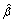with covariance matrix C = H-1. Since modes and stable points are the roots of the polynomial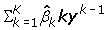we can estimate the variance of the root y* with the delta method,where the derivative of the root with respect to polynomial coefficients is calculated through the derivation of the implicit function, namely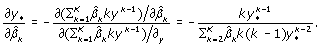(10)

The bimodality discovered has an important biological interpretation as an oxygen concentration in capillaries/blood vessels and brain matter. The saddle point may be used to discriminate PtO2 values of highly and normally oxygenated parts of the brain. As follows from our density estimation, the oxygen oncentration in brain matter is half that of the blood vessels with the dividing value (saddle point value) at about 37 mm Hg.

Although bimodality and consequently abnormality is visually obvious, we need a statistical support by hypothesis testing. Testing normality is equivalent to testing the null hypothesis H0 : α3 =α4 =α5 =α6 = 0. We test this hypothesis using the Wald and likelihood ratio test (ML Gauss-Hermite quadrature with 21 nodes is used in the following computations). Let C* denote a 4×4 covariance matrix of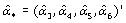as a sub matrix of the inverse to the Hessian, H-1. Then, using the Wald test, we have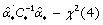, which gives the value 26 with the p-value less than 0.0001. For the likelihood ratio test, we compute 2(F2,min - F6,min), where F2,min and F6,min are minimal values of (5) with K = 2 and K = 6 respectively, which has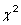(4) distribution. This test gives 2(F2,min -F6,min) = 37 with the p-value less that 0.0001. Thus, both tests confirm that the distribution of brain oxygen is not normal.

The advantage of the polynomial density estimation is obvious because it allows statistical hypothesis testing while the traditional kernel density estimation is used merely as the exploratory analysis. The former method of density estimation enables identifying the presence of two parts of the brain with statistically different levels of oxygen concentration.

Bump hunting: arsenic toenail distribution

Arsenic belongs to a group of toxic metals and may cause cancer. In particular, several papers relate the elevated concentration of arsenic in drinking water to bladder cancer by measuring the toenail arsenic concentration. Knowing the distribution of toenail arsenic is very important. For example, this distribution may help policy making to determine the threshold in the level of concentration above which the exposure to arsenic becomes dangerous for health. We hunt bumps at the right tail distribution .

Here we use data on toenail arsenic concentration in New Hampshire residents described in . The histogram of the toenail arsenic distribution based on n =1057 residents is 9 presented in Figure 2. The distribution is fairly close to lognormal. We show three density curves: normal, a nonparametric estimate with Gaussian kernel, and an exponential polynomial of the 10th degree. The latter density identifies a bump at right. Solving the polynomial equation of the ninth order, we find that the saddle point/threshold is -0.38. Using the delta-method with derivatives computed by formulas (10), we estimate the standard error of the threshold as 0.032.

Figure 2: Distribution of toenail srsenic on the log scale. The distribution of New Hampshire residents with elevated arsenic starts at 0.68 mg/1.

Summing up, a group of New Hampshire residents have an elevated arsenic toenail concentration, which starts from 0.68 ml/l. Approximately 0.76% of population have an arsenic concentration that is dangerous for health. Having that threshold one may identify the area of the state where the concentration of arsenic is above the limit.

#### Discussion

Modeling the log density via orthogonal polynomials can be viewed as an alternative to the gold standard kernel density estimation. The choice of the kernel, such as triangle or Gaussian, is less important but the choice of the bandwidth is critical: If the bandwidth is wide the density looks like normal if the bandwidth is narrow the density is jagged. Although some methods of the bandwidth selection, like cross-validation, have been suggested in the literature they are ad-hoc in nature, and merely used for exploratory distribution analysis. The adventure of the polynomial density estimation is that it is modelbased and therefore the classic statistical hypothesis testing applies. Consequently, we may rigorously answer the questions whether the distribution is normal or whether the discovered density bump is statistically significant. The log density estimation with orthogonal polynomials can be easily realized in modern statistical packages, such as S-Plus or R.

#### References

Select your language of interest to view the total content in your interested language

### Article Usage

• Total views: 12350
• [From(publication date):
November-2010 - Jul 17, 2019]
• Breakdown by view type
• HTML page views : 8557Can't read the image? click here to refresh